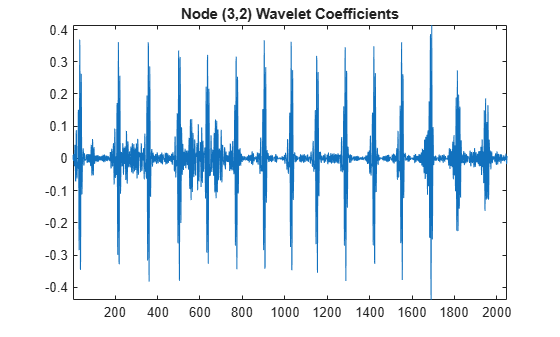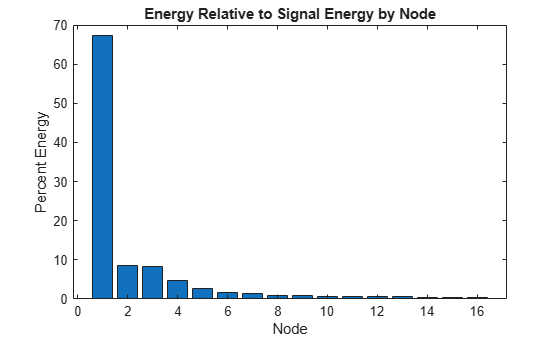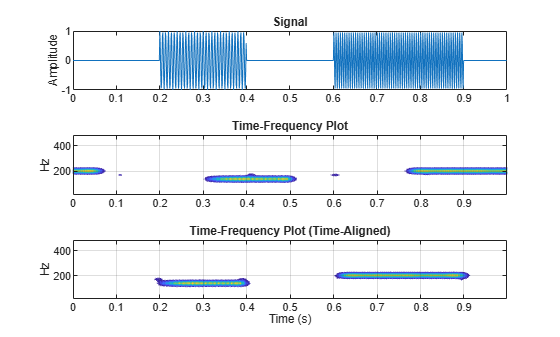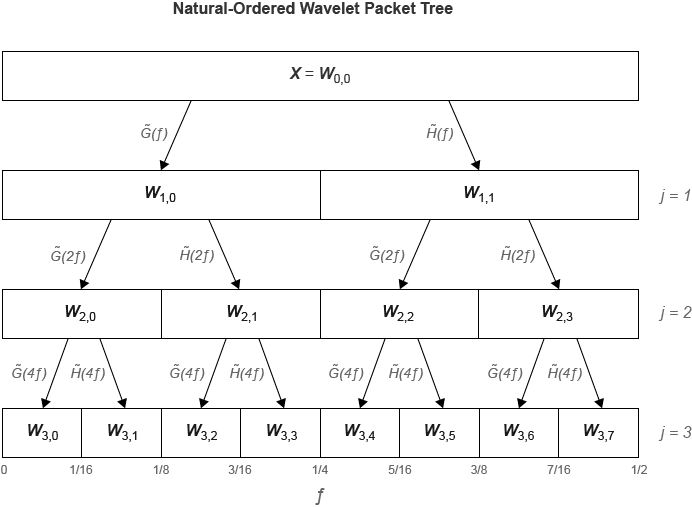# modwpt

Maximal overlap discrete wavelet packet transform

## Syntax

``wpt = modwpt(x)``
``wpt = modwpt(x,wname)``
``wpt = modwpt(x,lo,hi)``
``wpt = modwpt(___,lev)``
``````[wpt,packetlevs] = modwpt(___)``````
``````[wpt,packetlevs,cfreq] = modwpt(___)``````
``````[wpt,packetlevs,cfreq,energy] = modwpt(___)``````
``````[wpt,packetlevs,cfreq,energy,relenergy] = modwpt(___)``````
``[___] = modwpt(___,Name,Value)``

## Description

example

````wpt = modwpt(x)` returns the terminal nodes for the maximal overlap discrete wavelet packet transform (MODWPT) for the 1-D real-valued signal, `x`. NoteThe output of the MODWPT is time-delayed compared to the input signal. Most filters used to obtain the MODWPT have a nonlinear phase response, which makes compensating for the time delay difficult. This is true for all orthogonal scaling and wavelet filters, except the Haar wavelet. It is possible to time-align the coefficients with the signal features, but the result is an approximation, not an exact alignment with the original signal. The MODWPT partitions the energy among the wavelet packets at each level. The sum of the energy over all the packets equals the total energy of the input signal. The output of MODWPT is useful for applications where you want to analyze the energy levels in different packets.The MODWPT details (`modwptdetails`) are the result of zero-phase filtering of the signal. The features in the MODWPT details align exactly with features in the input signal. For a given level, summing the details for each sample returns the exact original signal. The output of the MODWPT details is useful for applications that require time-alignment, such as nonparametric regression analysis. ```

example

````wpt = modwpt(x,wname)` returns the MODWPT using the orthogonal wavelet filter specified by the `wname`.```

example

````wpt = modwpt(x,lo,hi)` returns the MODWPT using the orthogonal scaling filter, `lo`, and wavelet filter, `hi`.```
````wpt = modwpt(___,lev)` returns the terminal nodes of the wavelet packet tree at positive integer level `lev`. ```

example

``````[wpt,packetlevs] = modwpt(___)``` returns a vector of transform levels corresponding to the rows of `wpt`. ```
``````[wpt,packetlevs,cfreq] = modwpt(___)``` returns the center frequencies of the approximate passbands corresponding to the rows of `wpt`.```

example

``````[wpt,packetlevs,cfreq,energy] = modwpt(___)``` returns the energy (squared L2 norm) of the wavelet packet coefficients for the nodes in `wpt`.```

example

``````[wpt,packetlevs,cfreq,energy,relenergy] = modwpt(___)``` returns the relative energy for the wavelet packets in `wpt`.```

example

````[___] = modwpt(___,Name,Value)` returns the MODWPT with additional options specified by one or more `Name,Value` pair arguments.```

## Examples

collapse all

Obtain the MODWPT of an electrocardiogram (ECG) signal using the default length 18 Fejer-Korovkin (`'fk18'`) wavelet.

```load wecg; wpt = modwpt(wecg);```

`wpt` is a 16-by-2048 matrix containing the sequency-ordered wavelet packet coefficients for the wavelet packet transform nodes. In this case, the nodes are at level 4. Each node corresponds to an approximate passband filtering of $\left[n{f}_{s}/{2}^{5},\left(n+1\right){f}_{s}/{2}^{5}\right)$, where n = 0,...,15, and ${f}_{s}$ is the sampling frequency. Plot the wavelet packet coefficients at node (4,2), which is level 4, node 2.

```plot(wpt(3,:)) title('Node 4 Wavelet Packet Coefficients')```Obtain the MODWPT of Southern Oscillation Index data with the Daubechies extremal phase wavelet with two vanishing moments (`'db2'`).

```load soi; wsoi = modwpt(soi,'db2');```

Verify that the size of the resulting transform contains 16 nodes. Each node is in a separate row.

`size(wsoi)`
```ans = 1×2 16 12998 ```

Obtain the MODWPT of an ECG waveform using the Fejer-Korovkin length 18 scaling and wavelet filters.

```load wecg; [lo,hi] = wfilters('fk18'); wpt = modwpt(wecg,lo,hi);```

Obtain the MODWPT and full wavelet packet tree of an ECG waveform using the default length 18 Fejer-Korovkin (`'fk18'`) wavelet. Extract and plot the node coefficients at level 3, node 2.

```load wecg; [wpt,packetlevels,cfreq] = modwpt(wecg,'FullTree',true); p3 = wpt(packetlevels==3,:); plot(p3(3,:)) title('Level 3, Node 2 Wavelet Coefficients')```Display the center frequencies at level 3.

`cfreq(packetlevels==3,:)`
```ans = 8×1 0.0312 0.0938 0.1562 0.2188 0.2812 0.3438 0.4062 0.4688 ```

Obtain and plot the MODWPT energy and relative energy of an ECG waveform.

```load wecg [wpt,~,cfreq,energy,relenergy] = modwpt(wecg);```

Show that the sum of the MODWPT energies is equal to the sum of the energy in the original signal. The difference between the total MODWPT energy and the signal energy is small enough to be considered insignificant.

`disp(['Difference between MODWPT energy and signal energy: ',num2str(sum(energy)-sum(wecg.^2))])`
```Difference between MODWPT energy and signal energy: 3.6122e-09 ```

Plot the MODWPT energy by node.

```figure bar(1:16,energy) xlabel('Node') ylabel('Energy') title('Energy by Node')````disp(['Total power in passband: ',num2str(energy(1))])`
```Total power in passband: 200.8446 ```

Plot the relative energy and show the percentage of signal energy in the first passband [0,5.6250].

```figure bar(1:16,relenergy*100) xlabel('Node') ylabel('Percent Energy') title('Energy Relative to Signal Energy by Node')````disp(['Percentage of signal power in passband: ',num2str(relenergy(1)*100)])`
```Percentage of signal power in passband: 67.3352 ```

Obtain the time-aligned MODWPT of two intermittent sine waves in noise. The sine wave frequencies are 150 Hz and 200 Hz. The data is sampled at 1000 Hz.

```dt = 0.001; t = 0:dt:1-dt; x = cos(2*pi*150*t).*(t>=0.2 & t<0.4)+ sin(2*pi*200*t).*(t>0.6 & t<0.9); y = x+0.05*randn(size(t)); [wpta,~,Falign] = modwpt(x,'TimeAlign',true); [wptn,~,Fnon] = modwpt(x);```

Compare the nonaligned and time-aligned time-frequency plots.

```subplot(2,1,1); contour(t,Fnon.*(1/dt),abs(wptn).^2); grid on; ylabel('Hz'); title('Time-Frequency Plot (Nonaligned)'); subplot(2,1,2) contour(t,Falign.*(1/dt),abs(wpta).^2); grid on; xlabel('Time'); ylabel('Hz'); title('Time-Frequency Plot (Aligned)');```## Input Arguments

collapse all

Input signal, specified as a real-valued row or column vector. `x` must have at least two elements.

Data Types: `double`

Analyzing wavelet filter specified as a that corresponds to an orthogonal wavelet. If you specify the scaling (`lo`) and wavelet (`hi`) filters, `modwpt` ignores the `wname` input.

Valid orthogonal wavelet families begin with one of the following, followed by an integer, `N`, for example, `sym4`. Note, however, that `'haar'` is not followed by an integer.

• `'haar'` — Haar wavelet, which is the same as Daubechies wavelet with one vanishing moment, `'db1'`.

• `'dbN'` — Daubechies wavelet with `N` vanishing moments

• `'symN'` — Symlets wavelet with `N` vanishing moments

• `'coifN'` — Coiflets wavelet with `N` vanishing moments

• `'fkN'` — Fejer-Korovkin wavelet with `N` coefficients

To check if your wavelet is orthogonal, use `wavemngr('type',wname)` and verify that it returns `1` as the wavelet type. To determine valid values for N, use `waveinfo`, for example, `waveinfo('fk')`.

Scaling filter, specified as an even-length real-valued vector. `lo` must satisfy the conditions necessary to generate an orthogonal scaling function. You cannot specify both the scaling-wavelet filters and the `wname` input.

Wavelet filter, specified as an even-length real-valued vector. `hi` must satisfy the conditions necessary to generate an orthogonal wavelet. You cannot specify both the scaling-wavelet filters and the `wname` input.

Transform level, specified as a positive integer less than or equal to `floor(log2(numel(x)))`.

### Name-Value Arguments

Specify optional pairs of arguments as `Name1=Value1,...,NameN=ValueN`, where `Name` is the argument name and `Value` is the corresponding value. Name-value arguments must appear after other arguments, but the order of the pairs does not matter.

Before R2021a, use commas to separate each name and value, and enclose `Name` in quotes.

Example: `'Fulltree',true` returns the full wavelet packet tree

Option to return the full wavelet packet tree, specified as the comma-separated pair consisting of `'FullTree'` and either `false` or `true`. If you specify `false`, then `modwpt` returns only the terminal (final-level) wavelet packet nodes. If you specify `true`, then `modwpt` returns the full wavelet packet tree down to the specified level.

Example: `'Fulltree',true`

Option to time align wavelet packet coefficients with signal features, specified as the comma-separated pair consisting of `'TimeAlign'` and either `true` to time align or `false` to not align.

The scaling and wavelet filters have a time delay. Circularly shifting the wavelet packet coefficients in all nodes aligns the signal and wavelet coefficients in time. If you want to reconstruct the signal, such as by using `imodwpt`, do not shift the coefficients because time alignment is done during the inversion process.

Example: `'TimeAlign',true`

## Output Arguments

collapse all

Wavelet packet tree, returned as a matrix with each row containing the sequency-ordered wavelet packet coefficients. By default, `wpt` contains only the terminal level for the MODWPT. The default terminal level is either level 4 or `floor(log2(numel(x)))`, whichever is smaller. At level 4, `wpt` is a 16-by-`numel`(`x`) matrix. For the full tree, at level j, `wpt` is a 2j+2-2-by-`numel`(`x`) matrix, with each row containing the packet coefficients by level and index. The approximate passband for the nth row of `wpt` at level j is $\left[\frac{n-1}{{2}^{\left(j+1\right)}},\frac{n}{{2}^{\left(j+1\right)}}\right)$ cycles/sample, where n = 1,2,...2j.

Transform levels, returned as a vector. The levels correspond to the rows of `wpt`. If `wpt` contains only the terminal level coefficients, `packetlevs` is a vector of constants equal to the terminal level. If `wpt` contains the full wavelet packet table, `packetlevs` is a vector with 2j elements for each level, j. To select all the wavelet packet nodes at a particular level, use `packetlevs` with logical indexing.

Center frequencies of the approximate passbands in the `wpt` rows, returned as a vector. The center frequencies are in cycles/sample. To convert the units to cycles/unit time, multiply `cfreq` by the sampling frequency.

Energy of the wavelet packet coefficients for the `wpt` nodes, returned as a vector. The sum of the energies (squared L2 norms) for the wavelet packets at each level equals the energy in the signal.

Relative energy for each level, returned as a vector. The relative energy is the proportion of energy in each wavelet packet by level, relative to the total energy of that level. The sum of relative energies in all packets at each level equals 1.

## Algorithms

The `modwpt` performs a discrete wavelet packet transform and produces a sequency-ordered wavelet packet tree. Compare the sequency-ordered and normal (Paley)-ordered trees.Percival, D. B., and A. T. Walden. Wavelet Methods for Time Series Analysis. Cambridge, UK: Cambridge University Press, 2000.

 Walden, A.T., and A. Contreras Cristan. “The phase-corrected undecimated discrete wavelet packet transform and its application to interpreting the timing of events.” Proceedings of the Royal Society of London A. Vol. 454, Issue 1976, 1998, pp. 2243-2266.